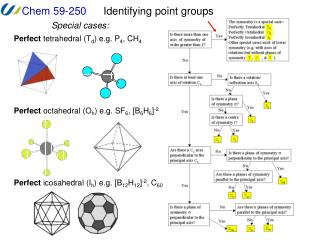DownloadDownload PresentationIdentifying point groups

# Identifying point groups

Download Presentation## Identifying point groups

- - - - - - - - - - - - - - - - - - - - - - - - - - - E N D - - - - - - - - - - - - - - - - - - - - - - - - - - -
##### Presentation Transcript

1. Identifying point groups Special cases: Perfect tetrahedral (Td) e.g. P4, CH4 Perfect octahedral (Oh) e.g. SF6, [B6H6]-2 Perfect icosahedral (Ih) e.g. [B12H12]-2, C60

2. Identifying point groups Low symmetry groups: Only* an improper axis (Sn) e.g. 1,3,5,7-tetrafluoroCOT, S4 Only a mirror plane (Cs) e.g. CHFCl2

3. Identifying point groups Low symmetry groups: Only an inversion center (Ci) e.g. (conformation is important !) No symmetry (C1) e.g. CHFClBr

4. Identifying point groups Cn type groups: A Cn axis and a h (Cnh) e.g. B(OH)3(C3h, conformation is important !) e.g. H2O2(C2h, conformation is important !) Note: molecule does not have to be planar e.g. B(NH2)3(C3h, conformation is important !)

5. Identifying point groups Cn type groups: Only a Cn axis (Cn) e.g. B(NH2)3(C3, conformation is important !) e.g. H2O2(C2, conformation is important !)

6. Identifying point groups Cn type groups: A Cn axis and a v (Cnv) e.g. NH3(C3v) e.g. H2O2(C2v, conformation is important !)

7. Identifying point groups Cn type groups: A Cn axis and a v (Cnv) e.g. NH3(C3v, conformation is important !) e.g. carbon monoxide, CO (Cv) There are an infinite number of possible Cn axes and v mirror planes. e.g. trans-[SbF4ClBr]-(C4v)

8. Identifying point groups Dn type groups: A Cn axis, n perpendicular C2 axes and a h (Dnh) e.g. BH3(D3h) e.g. NiCl4(D4h)

9. Identifying point groups Dn type groups: e.g. pentagonal prism (D5h) A Cn axis, n perpendicular C2 axes and a h (Dnh) e.g. Mg(5-Cp)2(D5h in the eclipsed conformation) View down the C5 axis e.g. square prism (D4h) e.g. carbon dioxide, CO2 or N2(Dh) There are an infinite number of possible Cn axes and v mirror planes in addition to the h.

10. Identifying point groups Dn type groups: A Cn axis, n perpendicular C2 axes and no mirror planes (Dn) -propellor shapes e.g. Ni(CH2)4(D4)

11. e.g. (SCH2CH2)3(D3 conformation is important!) e.g. propellor (D3) e.g. Ni(en)3(D3 conformation is important!) en = H2NCH2CH2NH2

12. Identifying point groups Dn type groups: A Cn axis, n perpendicular C2 axes and a d (Dnd) e.g. ethane, H3C-CH3 (D3d in the staggered conformation) dihedral means between sides or planes – this is where you find the C2 axes

13. e.g. Mg(5-Cp)2 and other metallocenes in the staggered conformation (D5d) View down the C5 axis These are pentagonal antiprisms e.g. square antiprism (D4d) e.g. allene or a tennis ball (D2d) e.g. triagular antiprism (D3d)

14. Identifying point groups We can use a flow chart such as this one to determine the point group of any object. The steps in this process are: 1. Determine the symmetry is special (e.g. tetrahedral). 2. Determine if there is a principal rotation axis. 3. Determine if there are rotation axes perpendicular to the principal axis. 4. Determine if there are mirror planes and where they are. 5. Assign point group.

15. NMR Spectroscopy and Symmetry This means that the number of signals in a spectrum tells provides the number of nuclei that are not related by symmetry and the relative intensity of each signal is proportional to the number of nuclei that are related to each other by symmetry. DE=(hn/2p)Bo h = Plank’s constant Bo = strength of the magnetic field n = frequency of signal (radio frequency) One type of spectroscopy that provides us structural information about molecules is Nuclear Magnetic Resonance (NMR) spectroscopy. An understanding of symmetry helps us to understand the number and intensity of signals we will observe. As with electrons, nuclei also have a spin quantum number, I. When I = 1/2, the possible values are +1/2 and -1/2. In a magnetic field, the nuclei have slightly different energies; we can measure this difference, E, to produce a spectrum. The actual E for a nucleus depends on the strength of the magnetic field and on its exact molecular environment so these are typically reported as a field-independent chemical shift, d. Since the differences in the energies of signals that are observed are very small, the chemical shift is reported in parts per million (ppm) with respect to a reference compound selected for each nucleus: d = [(nobs - nref)106] / nref. In practice, only atoms that are related by symmetry will have the same chemical shift.

16. NMR Spectroscopy and Symmetry This means that we can determine the symmetry of a molecule based on the number of signals that we see in the appropriate NMR spectrum (or vice versa). As an example, here are the number of signals that we would predict for the 19F NMR spectrum of a series of hexa-haloantimonate anions. [SbF6]- p.g.: Oh All F’s related 1 signal mer-[SbBr3F3]- p.g.: C2v 2 signals 2:1 intensity trans-[SbBr2F4]- p.g.: D4h All F’s related 1 signal [SbBrF5]- p.g.: C4v 2 signals 4:1 intensity cis-[SbBr2F4]- p.g.: C2v 2 signals 1:1 intensity fac-[SbBr3F3]- p.g.: C3v All F’s related 1 signal This is a gross oversimplification! NMR is one of the most powerful spectroscopic techniques and you will get much more detailed treatments in other classes. Read H&S 3.11 to get an idea of what other information is provided by multinuclear NMR spectroscopy (e.g. homonuclear and heteronuclear coupling, dynamic processes, etc.) that we will ignore. For the purposes of this course, we will assume that the structures of the molecules are static and that there is no coupling.